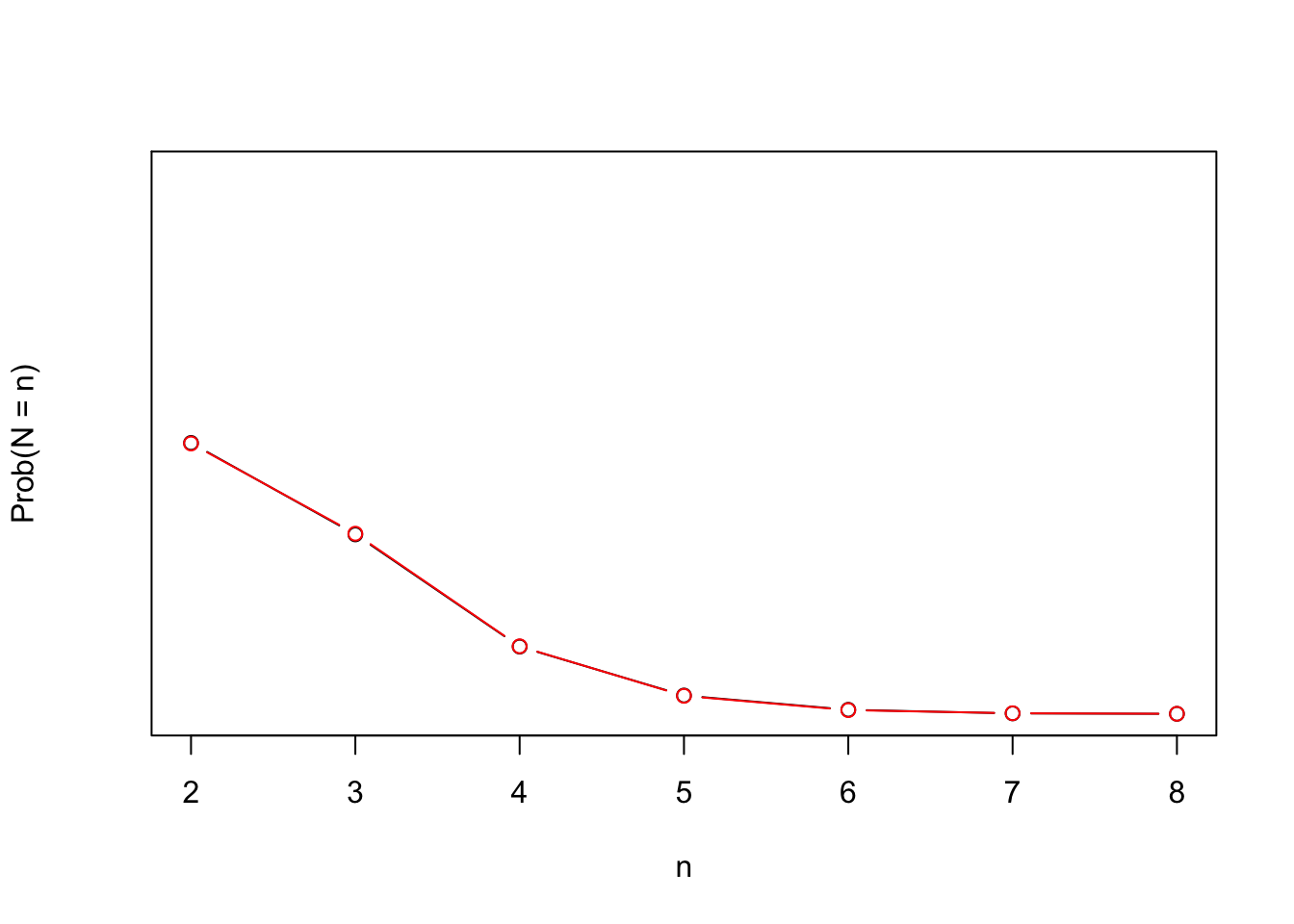# Sum of uniform random variables until sum is greater than one

math
Author

R package build

Published

November 10, 2022

## Introduction

I saw this problem on r/probabilitytheory

If you pick a uniformly random real number on [0,1] and repeat this until the sum of numbers picked is greater than 1, you’ll on average pick $$e \approx 2.718$$ numbers

Posed in a way that we can actually begin to solve this.

Sample independent uniform random variables $$U_1, U_2, \ldots$$ and let $$S_n = \sum_{i = 1}^n U_i$$. Let $$N$$ be the first integer $$n$$ such that $$S_n > 1$$. Find $$E[N]$$

Bonus question: What is the $$E[S_n]$$? Can we find its distribution?

Of course, the first step is to replicate the simple simulation.

## Simulation

# Sample from independent uniform U(0,1) distributions and stop when the sum
# is greater than 1. Let N be that first sum. Then E[N] = e

sim_sum <- function(t = 1) {
s_n <- 0
n <- 0
while(s_n < 1) {
s_n <- s_n + runif(1)
n <- n + 1
}
c(n = n, s_n = s_n)
}

reps <- 1e4
N_sim <- data.frame(t(replicate(reps, sim_sum())))
N_sim_table <- table(N_sim$n) N_sim_prob <- N_sim_table / reps K <- as.numeric(names(N_sim_table)) mean_N <- mean(N_sim$n)
plot(K, N_sim_prob, type = 'b', ylim = c(0, 1), ylab = 'P(N = n)', xlab = 'N')
abline(v = mean_N, lty = 2)
text(x = mean_N + 1, y = 0.75, sprintf('Mean(n) = %.03f', mean_N))## Proof

The idea of the proof is to directly solve:

$E[n] = \sum_{n = 1}^\infty n \cdot P(N = n)$

So we need to calculate, $$P(N = n)$$. This is the same as, $$P(S_{n - 1} \leq 1 \text{ and } S_n > 1)$$. If we calculate $$P(S_n \leq x) = F_{S_n}(x)$$ we can complete the proof.

### $$P(S_n \leq x)$$

Using the proof from this answer on math.stackexchange,

when $$x \in [0,1]$$, claim is that $$P(S_n \leq t) = \frac{x^n}{n!}$$. When $$n = 1$$, then $$P(S_1 \leq t) = P(U_1 \leq t) = t$$. Assume true for $$n$$. Then,

\begin{aligned} P(S_{n + 1} \leq x) &= P(S_n + U_{n + 1} \leq t)\\ &= \int_0^1 P(S_n + u \leq t) \underbrace{f(u)}_{1} ~du && S_n \text{ and } U_{n + 1} \text{ independent}\\ &= \int_0^1 P(S_n \leq t - u) ~du\\ &= \int_0^t \underbrace{P(S_n \leq t - u)}_{\text{Induction Hypothesis}} ~du && \text{Since } P(S_n \leq t - u) = 0 \text{ when } u \in [t, 1]\\ &= \int_0^t \frac{(t - u)^n}{n!} ~du \\ &= \frac{1}{n!} \left( \frac{-1}{n + 1} (t - u)^{n + 1}\Big|^{u = t}_{u = 0} \right)\\ &= \frac{t^{n + 1}}{(n + 1)!} \end{aligned}

### PMF of $$S_n$$

Since $$F_{S_n}(x) = \frac{x^n}{n!}$$ we have \begin{aligned} f_{S_n}(x) &= \frac{dF_{S_n}}{dx}\\ &= n \frac{x^{n - 1}}{n!} \end{aligned}

### $$P(N = n)$$

\begin{aligned} P(N = n) &= P(S_{n - 1} \leq 1 \text{ and } S_n > 1)\\ &= P(S_n > 1 | S_{n - 1} \leq 1) P(S_{n - 1} \leq 1)\\ &= \int_0^1 P(U_n + x > 1 | S_{n - 1} = x \leq 1) \underbrace{P(s \leq 1 | S_{n - 1} = s)}_{1} f_{S_{n - 1}}(x)~dx\\ &= \int_0^1 P(U_n + x > 1)~f_{S_{n - 1}}(x)~dx && U_n \text{ and } S_{n - 1} \text{ independent}\\ &= \int_0^1 (1 - F_n(1 - x))~f_{S_{n - 1}}(x)~dx\\ &= \int_0^1 x (n - 1)\frac{x^{n - 2}}{(n - 1)!}~dx\\ &= \frac{n - 1}{(n - 1)!} \int_0^1 x^{n - 1}~dx\\ &= \frac{n - 1}{n (n - 1)!} = \frac{n - 1}{n!} \end{aligned}

To double check the answer we can simulate

# This is formula we computed analytically
# (K - 1) / (factorial(K))
K <- as.numeric(names(N_sim_table))
expected_probs <- (K - 1) / (factorial(K))

plot(K, N_sim_prob, type = 'b', xlab = 'n', ylab = 'Prob(N = n)', ylim = c(0, 1), yaxp = c(0, 1, 4))
lines(K, expected_probs, type = 'b', col = 'red')### E[N] = e

\begin{aligned} E[N] &= \sum_{n = 2}^\infty n \cdot P(N = n)\\ &= \sum_{n = 2}^\infty \frac{n (n - 1)}{n!}\\ &= \sum_{n = 2}^\infty \frac{1}{(n - 2)!}\\ &= \sum_{n = 0}^\infty \frac{1}{n!} && \text{since } \sum_{n = 0}^\infty \frac{x^n}{n!} = e^x\\ &= e \end{aligned}